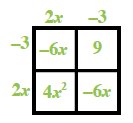### Home > CCA2 > Chapter Ch3 > Lesson 3.1.1 > Problem3-5

3-5.

For each of the following expressions, find at least three equivalent expressions. Which do you consider to be the simplest? Homework Help ✎

1. $(2x−3)^2+5$

Rewrite showing $(2x − 3)^2+5$ as a product.

$(2x − 3)(2x − 3)+5$

Multiply using a generic rectangle.Simplify the expression.

$(4x^2+(−6x)+(−6x)+9)+5\\(4x^2+(−12x)+9)+5$

Simplest Expression: $4x^2−12x+14$

1. $( \frac { 3 x ^ { 2 } y } { x ^ { 3 } } ) ^ { 4 }$

Start by writing the expression in the long form.

$\left(\frac{3\textit{x}^{2}\textit{y}}{\textit{x}^{3}}\right)\left(\frac{3\textit{x}^{2}\textit{y}}{\textit{x}^{3}}\right)\left(\frac{3\textit{x}^{2}\textit{y}}{\textit{x}^{3}}\right)\left(\frac{3\textit{x}^{2}\textit{y}}{\textit{x}^{3}}\right)$

Regroup using the Associative and Commutative properties of multiplication.

$\frac{3 \cdot 3 \cdot 3 \cdot 3 \cdot x^2 \cdot x^2\cdot x^2\cdot x^2 \cdot y \cdot y \cdot y \cdot y }{x^3 \cdot x^3 \cdot x^3 \cdot x^3 }$

$\frac{81\textit{y}^{4}}{\textit{x}^4}$